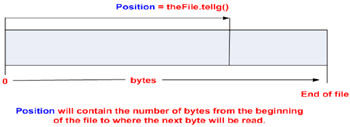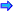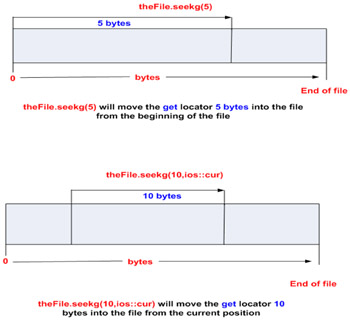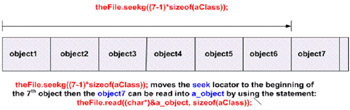# File Position Locators Functions

## File Position Locators & Functions

Each binary file has two file position locators that point to positions in the I/O file stream. One of these locators points to the location from where the next binary data will be read and the other locator points to the location to where the next binary data will be written.

The class methods: tellg() and tellp() return these locator values. The construct of these methods are:` streamposition tellg()   // used to find location of next input `and` streamposition tellp()   // used to find location of next output `These methods can be used for getting and putting data respectively. The streamposition is the byte location of the respective position locator.

For example:It is possible to move these file position locators using the methods: seekg() and seekp(). The output of these methods is based upon the data type: off_type which is an integer type defined by ios and the enumeration type: seekdir with the following enumerators:Open table as spreadsheet

Enumerator

Meaning

ios::beg

location at the beginning of the file

ios::cur

the current position of the position locator

ios::end

location at the end of the fileThe constructs of these methods are:` fstream &seekg(off_type offset, seekdir location = ios::beg); `and` fstream &seekp(off_type offset, seekdir location = ios::beg); `for moving the get and put file position locators respectively. The value offset is the distance in bytes the respective file locators will be moved from the value of location.

These two methods may be used as in the following examples:` theFile.seekg(5);                 // moves the get position locator to                                   // 5 bytes from the beginning of the file theFile.seekg(10, ios::cur);      // moves the get position locator to                                   // 10 bytes further into the file theFile.seekg(-5, ios::end);      // moves the get position locator to                                   // 5 bytes back from the                                   // end of the file `If the program was manipulating these locators for an object of class RealStuff, then the offset could be found as in the following:` offset = sizeof(RealStuff) * (recordNumber - 1) `Where offset would provide the byte location from ios::beg to where the object is to be the recordNumber object within the file.

For example the 1st object would be at record location 0 (i.e. 1 - 1) from ios::beg while the 10th object would be at record location 9 (i.e. 10 - 1) from ios::beg. Therefore` offset1 = sizeof(RealStuff) * (10 -1) `would store in offset the number of bytes from the beginning of the file to the beginning of the 10th object.

Using these functions, it is possible to locate particular objects: "records" within the file and to determine the number of objects: "records" within a binary file as in the following example:` theFile.seekg(0, ios::end);        // moves the get position locator // to the end of the file int position = theFile.tellg();    // determines how many bytes from the                                    // beginning of the file the get position                                    // locator is currently located int numberRecords = position/sizeof(aClass); // calculates the number of records `It is then possible to read from or to write to a particular record. For example to read the 7th record, code similar to the following could be written:` theFile.seekg((7-1)*sizeof(aClass)); theFile.read((char*)&a_object, sizeof(aClass)); `and the following could be used to write to the 19th record of the binary file:` theFile.seekp((19-1)*sizeof(aClass)); theFile.write((char*)&a_object, sizeof(aClass)); `Using these files with the constructors and the methods: open() and close(), it is possible to manage random access of binary files.

Using the following program: salsomny.cpp, run it on a week's amount of data. After you have done that, run the program: salsimnycount.cpp. Now run the two programs one more time and notice the differences.

Using the following program: salsomny.cpp run it on a week's data. After you have done that, run the program: salsimnysee.cpp. Next run the program: salsimnycount.cpp